## Place Value in Whole Numbers

### Learning Objectives

• Use place value to define all digits of a whole number

Our number system is called a place value system because the value of a digit depends on its position, or place, in a number. The number $537$ has a different value than the number $735$. Even though they use the same digits, their value is different because of the different placement of the $3$ and the $7$ and the $5$.

Money gives us a familiar model of place value. Suppose a wallet contains three $100 bills, seven$10 bills, and four $1 bills. The amounts are summarized in the image below. How much money is in the wallet?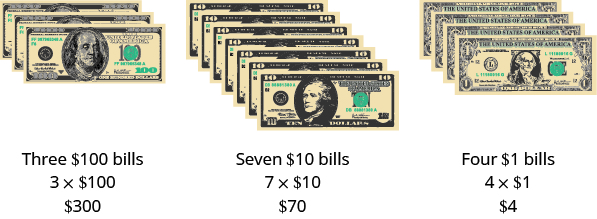Find the total value of each kind of bill, and then add to find the total. The wallet contains$374.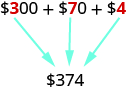Base-10 blocks provide another way to model place value, as shown in the image below. The blocks can be used to represent hundreds, tens, and ones. Notice that the tens rod is made up of $10$ ones, and the hundreds square is made of $10$ tens, or $100$ ones.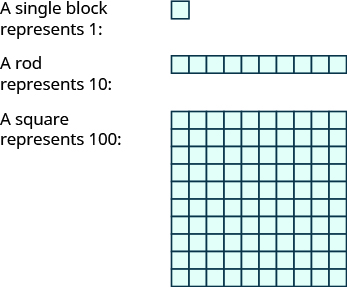The image below shows the number $138$ modeled with base-10 blocks.

We use place value notation to show the value of the number $138$.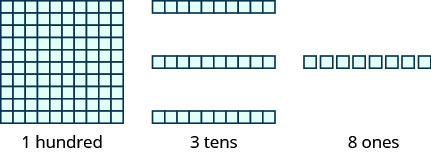Digit Place value Number Value Total value
$1$ hundreds $1$ $100$ $100\phantom{\rule{1 em}{0ex}}$
$3$ tens $3$ $10$ $30\phantom{\rule{1 em}{0ex}}$
$8$ ones $8$ $1$ $+\phantom{\rule{.5 em}{0ex}}8\phantom{\rule{1 em}{0ex}}$
$\text{Sum =}138\phantom{\rule{1 em}{0ex}}$

### example

Use place value notation to find the value of the number modeled by the base-10 blocks shown.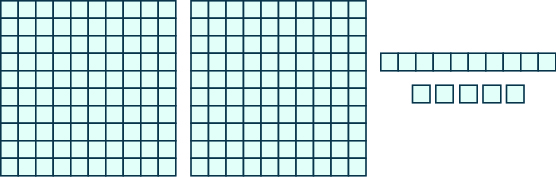### Try it

By looking at money and base-$10$ blocks, we saw that each place in a number has a different value. A place value chart is a useful way to summarize this information. The place values are separated into groups of three, called periods. The periods are ones, thousands, millions, billions, trillions, and so on. In a written number, commas separate the periods.

Just as with the base-$10$ blocks, where the value of the tens rod is ten times the value of the ones block and the value of the hundreds square is ten times the tens rod, the value of each place in the place-value chart is ten times the value of the place to the right of it.

The chart below shows how the number $5,278,194$ is written in a place value chart.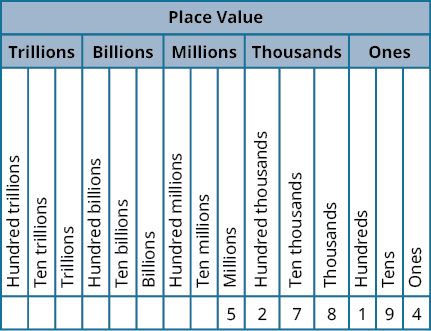• The digit $5$ is in the millions place. Its value is $5,000,000$.
• The digit $2$ is in the hundred thousands place. Its value is $200,000$.
• The digit $7$ is in the ten thousands place. Its value is $70,000$.
• The digit $8$ is in the thousands place. Its value is $8,000$.
• The digit $1$ is in the hundreds place. Its value is $100$.
• The digit $9$ is in the tens place. Its value is $90$.
• The digit $4$ is in the ones place. Its value is $4$.

### example

In the number $63,407,218$; find the place value of each of the following digits:

1. $7$
2. $0$
3. $1$
4. $6$
5. $3$

### try it

The video below shows more examples of how to determine the place value of a digit in a number.

## Contribute!

Did you have an idea for improving this content? We’d love your input.